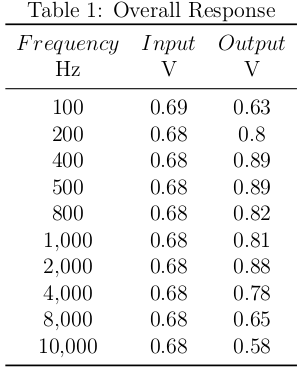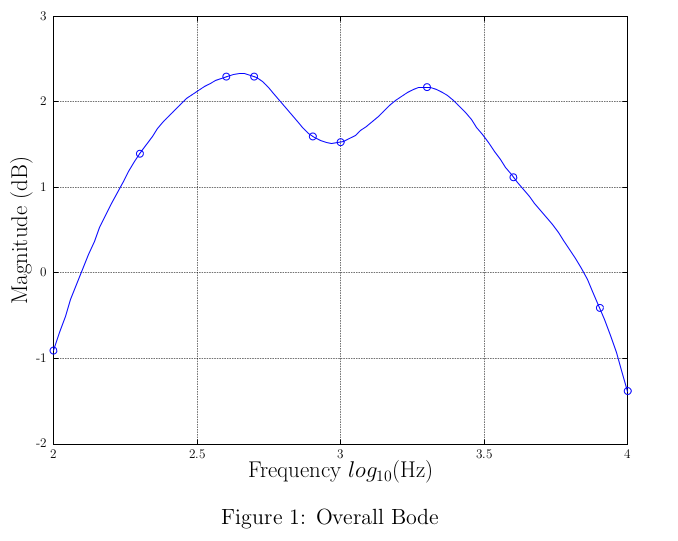# A Workflow for Creating Plots and Tables in LaTeX with Octave

This article describes a process of generating plots with Octave and including them into a LaTeX document seamlessly along with the associated data in a table. This worklow is particularly useful if you are working on a document with plots whose data may need to be revised. I have used this for lab reports successfully. This article also uses some parts from one of them as an example.

## Programs Used

• Octave
• A LaTeX renderer
• A shell or Make

## The Procedure

The data to be tabulated and plotted in LaTeX was

1. formatted into a comma-separated value file (CSV)
2. plotted with Octave
3. imported through LaTeX
4. automated with a shell script or a makefile

The materials used to demonstrate the procedure in this article can be found here in a zipped file. Here is a PDF rendering of the final LaTeX document.

### Formatting the Data

The data was formatted into a CSV to make importing into both LaTeX and Octave simple. Below are data points from measuring the response of a noise reduction system project of mine.

1.00E+002,0.6938,0.625
2.00E+002,0.6813,0.8
4.00E+002,0.6813,0.8875
5.00E+002,0.6813,0.8875
8.00E+002,0.6813,0.8188
1.00E+003,0.6813,0.8125
2.00E+003,0.6813,0.875
4.00E+003,0.6813,0.775
8.00E+003,0.6813,0.65
1.00E+004,0.6813,0.5812

### Plotting and Exporting to LaTeX with Octave

The data was processed and plotted in Octave. The "print" command with the '-dtex' arguement was used to export the plot as a LaTeX file along with other supporting files . In the Octave code below, I created a bode plot from input and output voltages across a spectrum of frequencies and then exported it. Octave created a .eps file to hold the plot graphics and a .tex to hold the text and axes formatting. Note that the x and y axes' labels (xlabel and ylabel in the code) are actually interpreted as LaTeX text, so I had complete control over formatting, such as text size and mathematical expressions.

clear;
hold off;

function plotter(source_file, gain_file)

% Frequencies, input V, output V
x = rot90(m(:, 1));
vi = rot90(m(:, 2));
vo = rot90(m(:, 3));
gain = vo ./ vi;  % compute gain

% Convert to base 10 log scales
x = log10(x);
gain = 20*log10(gain);

% Fit spline to data
xx = linspace(min(x), max(x), 100);
yy = spline(x,gain,xx);

% Plot
hold off;
plot(x, gain, 'o');
hold on;
plot(xx,yy);

xlabel('\LARGE Frequency $$log_{10}(\si{\hertz})$$');
ylabel('\LARGE Magnitude (\si{\decibel})');
grid on;

% Export
print(gain_file, '-dtex');
end

plotter('overall.csv', 'overall.tex');

### Importing a CSV file into a LaTeX Table

I used the "pgfplotstable" package to include and typeset the table . The "booktabs" package was included for the horizontal rules, and the "siunitx" package was included for S.I. unit formatting. The following table was contructed with only horizontal rules for a clean look.

\begin{table}[H]
\begin{center}
\caption{Overall Response}
\label{tab:overall}
\pgfplotstabletypeset
[
col sep=comma,
multicolumn names,
display columns/0/.style={column name=$$Frequency$$},
display columns/1/.style={column name=$$Input$$},
display columns/2/.style={column name=$$Output$$},
{
before row=\toprule,
after row={\si{\hertz} & \si{\volt} & \si{\volt}\\ \midrule},
},
every last row/.style={after row=\bottomrule}
]{overall.csv}
\end{center}
\end{table}Figure 1. A table imported from CSV

### Importing an Octave-generated Plot into LaTeX

The plot generated by Octave was included as a typical figure in LaTeX. Note that the .eps is included by the .tex file. In the following code, I scale the plot to 60%, caption it, and then label it for references.

\begin{figure}[H]
\centering
\scalebox{0.6}{\input{overall.tex}}
\caption{Overall Bode}
\label{fig:exoverall}
\end{figure}Figure 2. A plot imported from Octave

### Automating the Workflow with a Shell

When the included data in the document is modest, one can simply use a shell command and maybe even take the extra effort of putting it into a script file. A single line will do. Note that the double compile is necessary for LaTeX to link the inline references. The double ampersands prevent further execution if the previous instruction has failed.

octave plots.m && pdflatex bode.tex && pdflatex bode.tex

### Advance Workflow Automation with Make

Suppose the aforementioned one-line command is inadequate, since one wants to compile quickly to see small changes in a "live" manner. If the number of graphs is significant, Octave's slow plotting and exporting will slow down the workflow. To solve this, I wrote a simple makefile to conditionally plot the graphs and compile the document.

bode.pdf: overall.tex bode.tex
pdflatex bode.tex && pdflatex bode.tex

overall.tex: plots.m overall.csv
octave plots.m

clean:
rm -f bode.aux bode.log bode.pdf overall-eps-converted-to.pdf \
overall.eps overall.tex

The original implementation of the above makefile also ran my SPICE simulations and formatted the data into CSV files before plotting and including the results into the LaTeX document. The makefile allows one to write their document as they work on the data.

### A Warning on Automation

Automated generation of files usually means that your working directory will become a mess. Protect your files by including a way to remove the generated files (perhaps with the "make clean") and by creating directories to organize your critical files. One should also take care to not accidentally delete important files with lazy regular expressions as I have.

## Notes

Written on the 30th of October in 2014